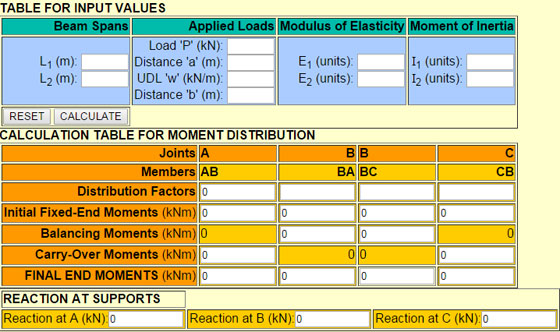# Moment Distribution Calculator and its functionalitiesMoment Distribution Calculator is useful for Indeterminate beam having two spans. Left span contains a point load and right span contains fractional UDL commencing from intermediate support.

Note : Put the value of moments of inertia of both the spans in the similar units. If I1 is identical to twice of I2, just put I1=2 and I2=1. Likewise the Modulus of Elaticity for both the spans belong in the identical units. If E1 is corresponding with twice of E2, just put E1=2 and E2=1.

Go through the following link to use the calculator Moment Distribution Calculator for Indeterminate beam of two spans